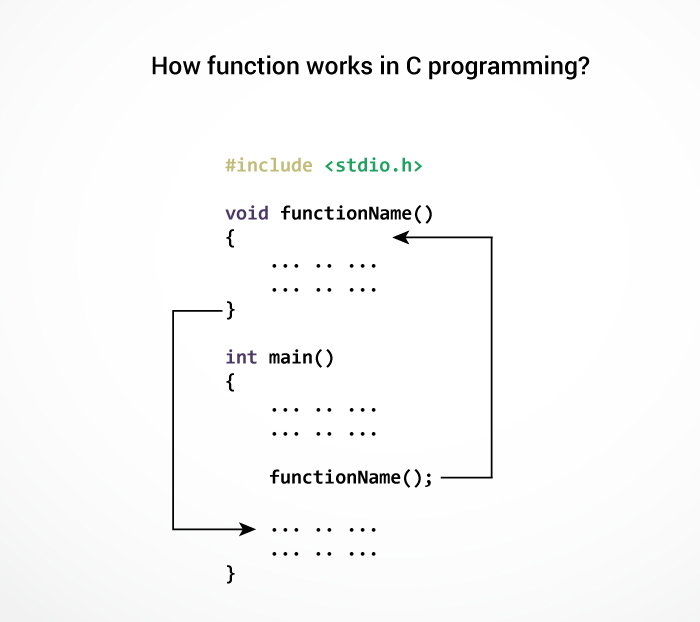C Functions

# C Functions

#### In this tutorial, you will be introduced to functions (both user-defined and standard library functions) in C programming. Also, you will learn why functions are used in programming.

A function is a block of code that performs a specific task.

Suppose, you need to create a program to create a circle and color it. You can create two functions to solve this problem:

• create a circle function
• create a color function

Dividing a complex problem into smaller chunks makes our program easy to understand and reuse.

## Types of function

There are two types of function in C programming:

### Standard library functions

The standard library functions are built-in functions in C programming.

These functions are defined in header files. For example,

• The `printf()` is a standard library function to send formatted output to the screen (display output on the screen). This function is defined in the `stdio.h` header file.
Hence, to use the `printf() `function, we need to include the `stdio.h` header file using `#include <stdio.h>`.
• The `sqrt()` function calculates the square root of a number. The function is defined in the `math.h` header file.

### User-defined function

You can also create functions as per your need. Such functions created by the user are known as user-defined functions.

## How user-defined function works?

```#include <stdio.h>
void functionName()
{
... .. ...
... .. ...
}

int main()
{
... .. ...
... .. ...

functionName();

... .. ...
... .. ...
}
```

The execution of a C program begins from the `main()` function.

When the compiler encounters `functionName();`, control of the program jumps to

` void functionName()`

And, the compiler starts executing the codes inside `functionName()`.

The control of the program jumps back to the `main()` function once code inside the function definition is executed.Note, function names are identifiers and should be unique.

This is just an overview of user-defined functions. Visit these pages to learn more on: